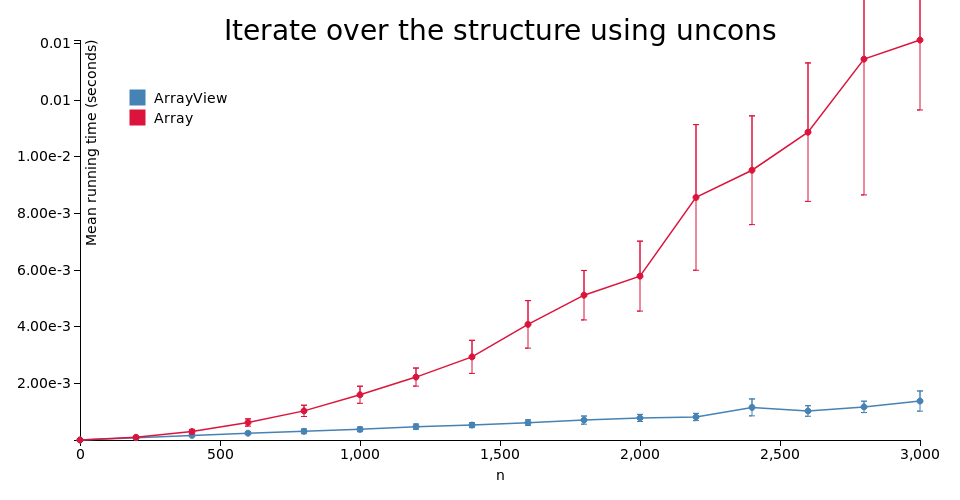Package

purescript-array-views

Repository
klntsky/purescript-array-views
BSD-3-Clause
klntsky
Published on
2019-05-01

Time complexity of `uncons` called on `Array` is O(n) because `.slice()` is used to preserve purity. Thus iterating over `Array` using `uncons` is O(n2).

But since purescript `Array`s are persistent at runtime, it is possible to defer multiple `.slice()` calls by introducing another data structure and operating on it instead.

`ArrayView` contains a reference to some `Array` coupled with two numbers: index where the view starts relative to the beginning of the array and the length of the view.

`newtype ArrayView a = View { from :: Int, len :: Int, arr :: Array a }`

So, instead of slicing, it is possible to just shift the indices.

Obviously, this technique does not improve the asymptotics of `cons`/`snoc`/`append` and other array constructing functions, so if the code uses these, there will be no benefit in replacing `Array` with `ArrayView`.

It should be noted once more that iterating over ordinary arrays using `cons` and `uncons`, which is common in Haskell (with lists) is a bad practice. Often such code can be refactored to use some type of fold and/or other standard functionals. However, there are cases where it is not straightforward, or where using `uncons`/`unsnoc` is more syntactically appealing, e.g. when iterating over many structures at once.

Asymptotics comparison

For every function in `Data.Array` there is a corresponding function in `Data.ArrayView`, though most of them are plain reuses up to conversions between `Array` and `ArrayView`. Those with different time complexities are listed below:

functions Array / NonEmptyArray ArrayView / NonEmptyArrayView Note
`slice`, `uncons`, `unsnoc`, `tail`, `init`, `take`, `drop`, `takeEnd`, `dropEnd` O(n) O(1) n is the length of the resulting array
`span` (used by `takeWhile`, `dropWhile`) O(n+m) O(n) n is the length of the `init` array, m is the length of the `rest`
`Data.ArrayView.toArray` - O(n) O(1) if the given view corresponds to the whole array
`Data.ArrayView.fromArray` O(1) -

API

This package's API mimics the API of `purescript-arrays` up to certain extent. For most use cases, just changing the imports is enough.

This table may be useful for incorporating this library into existing codebase:

Name Replacement Note
`Data.Array` `Data.ArrayView`
`Data.Array.NonEmpty` `Data.ArrayView.NonEmpty`
`Data.Array.NonEmpty.fromArray` `Data.ArrayView.NonEmpty.fromArrayView`
`Data.Array.NonEmpty.toArray` `Data.ArrayView.NonEmpty.toArrayView`
`Data.Array.some`, `many` `Data.ArrayView.some`, `many` `Lazy (f (Array a))` constaint is not changed to `Lazy (f (ArrayView a))` because of `OrphanInstances`
`Data.Array.NonEmpty.appendArray` `Data.ArrayView.NonEmpty.appendArrayView`

Impact on GC

Since every `ArrayView` holds a reference to some array, the latter can't be garbage-collected while the former is used. This leads to a memory consumption overhead.

If you need to free unused parts of array, use `Data.ArrayView.force :: forall a. ArrayView a -> ArrayView a` (which performs slicing and therefore is O(n)).

Benchmarks